## Soil Mechanics and Asphalt Testing

BTRA Soil Mechanics Laboratory is ready to undertake the following tests for soil classification as per Bureau of Indian Standard Test Methods.
1. Specific Gravity (IS 2720 Part-3)

2. Particle Size Analysis (dry/wet) (IS 2720 Part-4)

3. Liquid Limit (IS 2720 Part-5)

4. Plastic Limit (IS 2720 Part-5)

5. Shrinkage Limit (IS 2720 Part-6)

6. Natural Moisture Content (IS 2720 Part-9)

7. Differential / Free Swell Index (IS 2720 Part-40)

Apart from soil classification tests as mentioned above, other following tests are also undertaken.

8. Standard Proctor Compaction Test (IS 2720 Part-7)

9. Modified Proctor Compaction Test (IS 2720 Part-8)

10. Unconfined Compressive Strength (IS 2720 Part-10)

11. Triaxial Shear Test (IS 2720 Part-11)

12. Direct Shear Test (IS 2720 Part-13)

13. California Bearing Ratio (CBR) Test (IS 2720 Part-16)

## TESTS ON AGGREGATE

S. No. Specified Test Perform Test Method
1. Water content IS 13030: 1991 (RA 2016)
2. Specific gravity of aggregate IS 2386 part 3 (RA 2016)
3. Density of aggregate IS 2386 part 3 (RA 2016)
4. Sieve analysis IS 2386 part 1 (RA 2016)
5. Flakiness Index IS 2386 part 1 (RA 2016)
6. Elongation Index IS 2386 part 1 (RA 2016)
7. Angularity number IS 2386 part 1 (RA 2016)
8. Impact value IS 2386 (part 4): 1963
9. Abrasion value IS 2386 (part 4): 1963

## TESTS ON ASPHALT

S. No. Material Test name Test Method
1. Asphalt/bitumen Ductility IS 73 2013
Saybolt viscosity IS 73 2013
Flash and fire point IS 73 2013
Softening point IS 73 2013
Penetration test IS 73 2013
Cloud & pour point apparatus IS 73 2013
Carbon Residue apparatus IS 73 2013
2. Bituminous mixtures Marshal mix design ASTM D6927
Tensile strength ratio AASHTO T 283
Wheel rutting ASTM D8292
Asphalt/bitumen extraction ASTM D2172
3. Field tests Light weight deflectometer (LWD) ASTM D2583
Asphalt pavement field density Pavetracker (NDT) ASTM D7113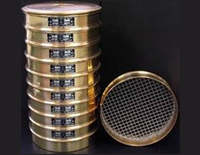## 1) PARTICLE SIZE ANALYSIS (IS 2720 PART-4)

The analysis of soil by particle size provides a useful engineering classification system from which a considerable amount of empirical data can be obtained.

## 2) LIQUID LIMIT (IS 2720 PART-5)

The liquid limit is defined as the moisture content at which the soil passes from plastic limit to liquid limit. It is very much helpful for the classification properties of the soil materials. The liquid limit gives a measure of the shearing resistance of the soil, when mixed with water.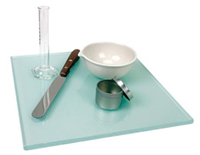## 3) PLASTIC LIMIT (IS 2720 PART-5)

The plastic limit of soil is the water content of the soil below which it ceases to be a plastic. It starts to crumble when rolled into threads of 3mm dia.

## 4) SPECIFIC GRAVITY (IS 2720 PART-3)

It is the ratio of the weight of a given volume of a material in air at a standard temperature to the weight in air of an equal volume of distilled water at the same stated temperature.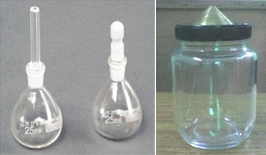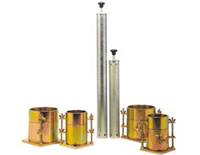## 5) STANDARD AND MODIFIED PROCTOR COMPACTION TEST (IS 2720 PART-7 & 8)

Soil compaction is a fundamental requirement for the construction of earth fill for dams, reservoirs, canal embankments, highway, railway and runways. The relationship between soil moisture content and compacted dry density is very much useful for deciding construction specification and quality control of compacted earth fill.

## 6) UNCONFINED COMPRESSIVE STRENGTH (IS 2720 PART-10)

Determination of soil shear strength is essential for designing the foundation of the structure, retaining wall, soil slopes and so on.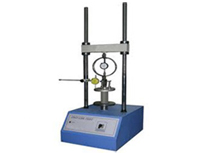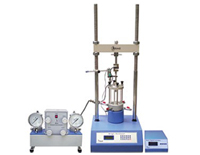## 7) TRIAXIAL SHEAR TEST (IS 2720 PART-11)

The knowledge of stress- strain and strength characteristics of the soil is of primary importance for the analysis and design of earth and earth retaining structures and foundations. Triaxial test are performed to simulate different types of stress and drainage conditions that can occur in the sub-soil for simulating the effect of building construction, excavation, tunneling etc.

## 8) DIRECT SHEAR TEST (IS 2720 PART-13)

It is carried out with an apparatus consisting of a square divided into two halves. The specimen, contained in a box is subjected to a constant normal load while an increasing horizontal force is applied to the shear box.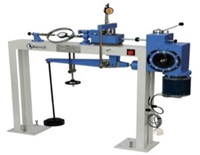## 9) CALIFORNIA BEARING RATIO (CBR) (IS 2720 PART-16)

To determine the relative bearing ratio and expansion characteristics under known surcharge weight for the design of road, pavement, and runways. The CBR test is used for the selection of materials and control of subgrade.